+

# Writing an Equation

Author: Sophia Tutorial
##### Description:

Given a real-world scenario, identify an equation to solve the scenario

(more)

Sophia’s self-paced online courses are a great way to save time and money as you earn credits eligible for transfer to many different colleges and universities.*

No credit card required

37 Sophia partners guarantee credit transfer.

299 Institutions have accepted or given pre-approval for credit transfer.

* The American Council on Education's College Credit Recommendation Service (ACE Credit®) has evaluated and recommended college credit for 32 of Sophia’s online courses. Many different colleges and universities consider ACE CREDIT recommendations in determining the applicability to their course and degree programs.

Tutorial
what's covered
This tutorial covers writing an equation, through the discussion of:
1. Solving an Equation: A Review
2. Writing an Equation

## 1. Solving an Equation: A Review

To review, an equation is a mathematical statement that two expressions are equal or have the same value. When solving an equation, you want to determine or solve for the value of the variable or unknown quantity. The first step in solving an equation is to combine like terms on either side of the equation. You can then use inverse operations to undo operations in the equation to isolate the variable.

## 2. Writing an Equation

There are several crucial steps in writing an equation to represent a situation:

1. Avoid skimming the problem. Read the entire problem to avoid missing or misinterpreting any information.
2. Determine what the question is asking you to find. These unknown quantities will help identify and define the variables in the equation.
3. Clearly state the letter that will be used for the variable and what that variable represents in the context of the problem. The letter x is commonly used as a variable, but any letter or non-numeral symbol can be used to represent an unknown quantity.
4. Use the variables and other necessary given quantities from the situation to write an equation that represents the problem.
5. Review the equation to make sure it is logical and accurate.

### IN CONTEXT

For her cellular phone service, Seylon pays \$32 a month plus \$0.75 for each minute over the allowed minutes in her plan. Seylon received a bill for \$47 last month. How many minutes did she use her phone beyond the allowed minutes?

You want to know how many minutes she used over the allowed minutes, so this unknown quantity will be your variable. Therefore you can define x as the number of minutes used over her allowance.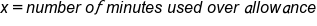Using the given information and a variable, you know that Seylon pays \$32 per month plus an additional \$0.75—or 0.75 dollars—for every minute used over the allowance. Therefore, you will need to multiply 0.75 times your variable, x. You also know that 32 plus 0.75x will equal the total amount paid, \$47. It follows, then, that your equation is: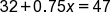To solve this equation, you want to isolate your x variable. Start by subtracting 32 from each side of the equation, which simplifies the expression to: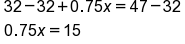Next, divide by 0.75 on both sides of the equation, which provides the solution of x equals 20.This means that Seylon used 20 minutes over her allowance, which seems like a reasonable solution, as it’s not a negative number or an excessively big number. You can verify that your solution is correct by substituting it back into the original equation. This translates to: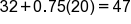Using the order of operations, you can simplify the left side of the equation, starting with multiplication and moving on to addition. 0.75 times 20 equals 15, plus 32, equals 47. Therefore, your equation is 47 equals 47. This is a true statement, which means your solution of x equals 20 is correct.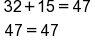try it
Suppose the population of Zimbabwe in 2003 was about 12.6 million, which was 1 million more than four times the population in 1950. How would you determine the population of Zimbabwe in 1950? You want to find the population in 1950, so that will be your variable. Let x equal the population of Zimbabwe in 1950, and you can define this in millions of people, which will make your equation simpler to write.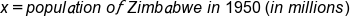Using your given information, you know that the population was 12.6 million in 2003, which was 1 million more than four times the population in 1950.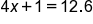To solve this equation and isolate the x variable, start by subtracting 1 on both sides. This simplifies to the expression 4x equals 11.6. Next, divide by 4 on each side of the equation, which simplifies to x equals 2.9. This means that the population of Zimbabwe was 2.9 million in 1950, which, again, seems like a reasonable solution. It’s not too big or small compared to 12.6 million, and the answer is not negative.You can verify that the solution is correct by substituting it back into your original equation. Simplifying using the order of operations arrives at the equation 12.6 equals 12.6, which is a true statement. Your solution is correct.summary
Today you reviewed how to solve an equation. You also learned the crucial steps involved in writing an equation that represents a situation: avoid skimming, determine what the question is asking you to find, clearly state the letter that will be used for the variable, use the variables and other necessary given information to write an equation that represents the problem, and, finally, review the equation to make sure it is logical and accurate.

Source: This work is adapted from Sophia author Colleen Atakpu.

Rating